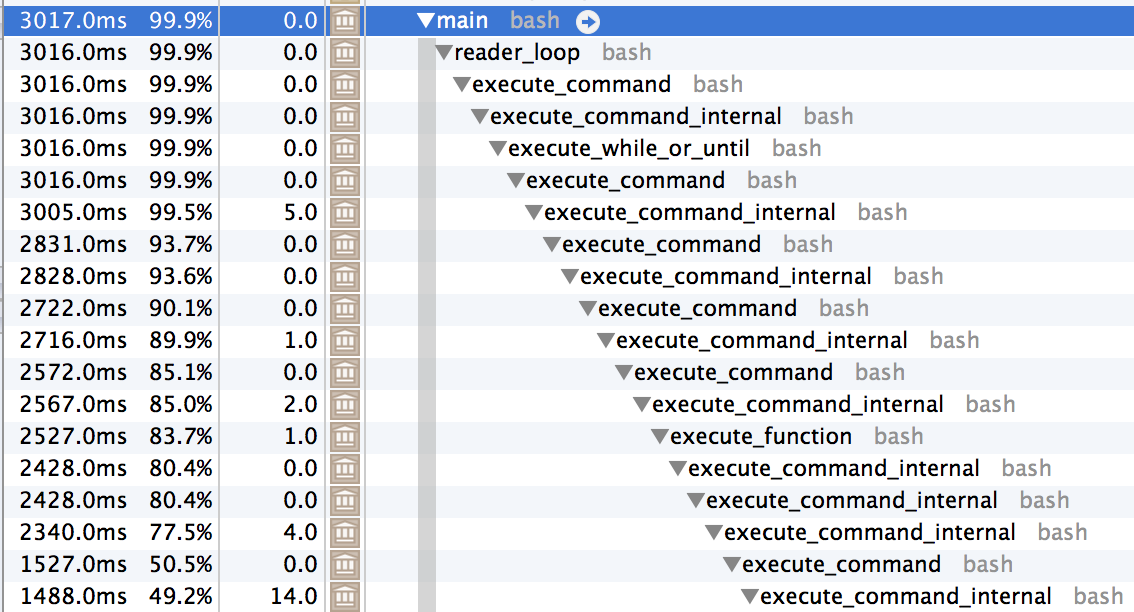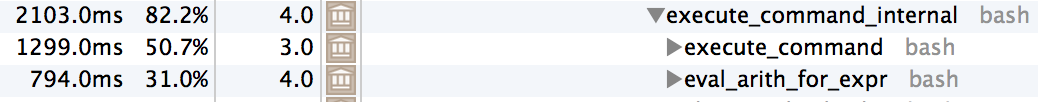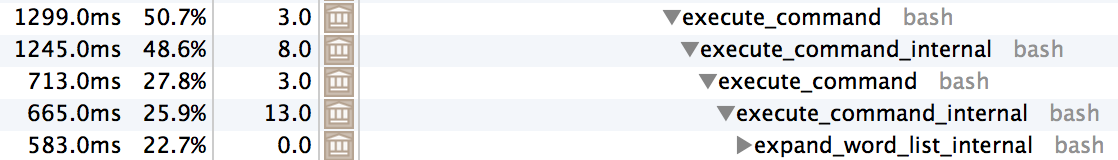# Bash Performance Tricks

My coworkers presented a silly programming interview style question to me the other day: given a list of words, find the largest set of words from that list that all have the same hash value. Everyone was playing around with a different language, and someone made the claim that it couldn't be done efficiently in bash. Rising to the challenge, I rolled up my sleeves and started playing around.

The first trick was to figure out how to write the hash function in bash. bash has functions, but they can only return an exit status in the range 0-255. There are a couple of different ways to do that, but I opted to return the value in a global variable. We also want to iterate through the letters of the word and want to take great care not invoke another process while doing so (so while read letter; do math; done <(grep -o <<<$word) is out of the question). Instead, we will use a for loop with bash expansions to iterate of each character. Finally, we will use bash 4.0 associative arrays map a letter to its corresponding index (for computing hash values). # We will return into this variable. declare -i HASH_RESULT function kr1 { local word=$1
HASH_RESULT=0
for (( i = 0; i <${#word}; i++)); do local letter=${word:$i:1} (( HASH_RESULT += letter_value[$letter] ))
done
}


Full program source below1. With the hash function implemented, it is fairly straightforward to finish the rest of the program:

while read word; do
kr1 $word (( hash_to_count[$HASH_RESULT]++ ))
hash_to_words[$HASH_RESULT]+="$word"

if (( hash_to_count[$HASH_RESULT] > max_count )); then max_count=${hash_to_count[$HASH_RESULT]} max_hash=$HASH_RESULT
fi
done <word.lst
echo ${hash_to_words[$max_hash]}


At this point it became interesting. My bash solution outperformed all the other bash solutions by a fair margin, but I wanted to see if I could do better. I ran it under a profiler and saw that it was spending all its time in many nested layers of execute_command.This gave me the idea to try inlining the function call. Quickly prototyping a variation using an inlined function call, I run some trials (and collect statistics with my favorite tool, histogram.py2):

for variation in hash.bash hash.bash.inlined; do
echo $variation for trial in {1..30}; do start=$EPOCHREALTIME
bash $variation > /dev/null echo$((EPOCHREALTIME - start))
done | histogram.py --confidence=.90 | head -n 2
echo
done
hash.bash
# NumSamples = 30; Min = 3.43; Max = 3.99
# Mean = 3.529906 (+/- 0.028584); Variance = 0.009060; SD = 0.095184; Median 3.509426

hash.bash.inlined
# NumSamples = 30; Min = 2.84; Max = 3.16
# Mean = 2.932449 (+/- 0.016860); Variance = 0.003152; SD = 0.056141; Median 2.917874


As you can see, there is a greater than 15% improvement gain from inlining the function! We take this approach further, removing the local variable letter and making our code compact:

for (( i = 0; i <${#word}; i++)); do (( HASH_RESULT += letter_value[${word:$i:1}] )) done  Running with this variation, we see yet another significant improvement: hash.bash.inline_nolocals # NumSamples = 30; Min = 2.69; Max = 2.84 # Mean = 2.749286 (+/- 0.010406); Variance = 0.001201; SD = 0.034651; Median 2.746643  At this point we run again under a profiler and notice something interesting: the first time the runtime of an execute_command call isn't dominated by another recursive call to execute_command, the function eval_arith_for_expr consumes a large portion of the time.Furthermore, we see that a large portion of the rest of the time is eventually spent in expand_word_list_internal:These observations lead us to another technique - we will use only one character variable names to try to optimize for these two functions. Running again with all of these optimizations, we get a huge performance improvement: hash.bash.one_char_names # NumSamples = 30; Min = 2.33; Max = 2.44 # Mean = 2.371499 (+/- 0.008031); Variance = 0.000715; SD = 0.026743; Median 2.363547  We can take this further, but I think I'm going to quit here for now - I improved performance by almost 50% by using a profiler and some bash-foo. Final program below3. One final note - for the love of all that is holy, don't write performant programs in bash! 1. Initial program. #!/usr/bin/env bash if ((BASH_VERSINFO < 4)); then echo "Sorry, you need at least bash-4.0 to run this script." >&2 exit 1 fi # An associate array mapping each letter to its index. declare -A letter_value i=97 # ascii 'a'. for letter in a b c d e f g h i j k l m n o p q r s t u v w x y z; do letter_value[$letter]=$((i++)) done # We will return into this variable. declare -i HASH_RESULT function kr1 { local word=$1
HASH_RESULT=0
for (( i = 0; i <${#word}; i++)); do local letter=${word:$i:1} (( HASH_RESULT += letter_value[$letter] ))
done
}

declare -a hash_to_count
declare -a hash_to_words

declare -i max_count=0
declare -i max_hash=-1

kr1 $word (( hash_to_count[$HASH_RESULT]++ ))
hash_to_words[$HASH_RESULT]+="$word"

if (( hash_to_count[$HASH_RESULT] > max_count )); then max_count=${hash_to_count[$HASH_RESULT]} max_hash=$HASH_RESULT
fi
done <word.lst

echo ${hash_to_words[$max_hash]}


2. Here I'm using a modified version of bitly/data_hacks that includes the flag --confidence specifying a confidence interval around the mean to report.

3. Final program.

#!/usr/local/bin/bash

if ((BASH_VERSINFO < 4)); then
echo "Sorry, you need at least bash-4.0 to run this script." >&2
exit 1
fi

# An associate array mapping each letter to its index.
declare -A l
i=97  # ascii 'a'.
for letter in a b c d e f g h i j k l m n o p q r s t u v w x y z; do
l[$letter]=$((i++))
done

declare -a c
declare -a v

declare -i m=0
declare -i n=-1

for (( i = 0; i <${#w}; i++)); do (( h += l[${w:$i:1}] )) done (( c[$h]++ ))
v[$h]+="$w"
if (( c[$h] > m )); then m=${c[$h]} n=$h
echo ${v[$n]}